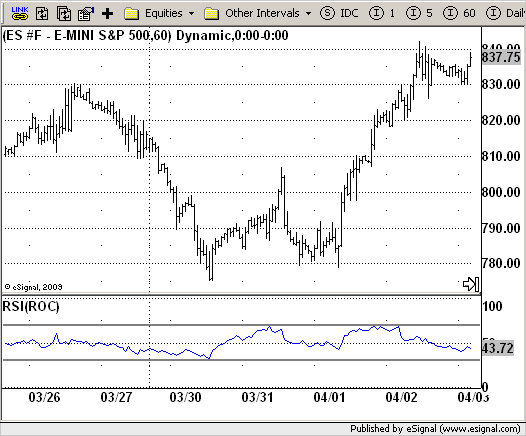# RSI based on ROC

ICE Data Services -

 RSI_ROC.efs EFSLibrary - Discussion Board

File Name: RSI_ROC.efs

Description:
RSI based on ROC

Formula Parameters:
Length of RSI : 20
Length of ROC : 20
Source of ROC : Close
Upper : 70
Lower : 30

Notes:
This is the new-age indicator which is version of RSI calculated upon
the Rate-of-change indicator.
The name "Relative Strength Index" is slightly misleading as the RSI
does not compare the relative strength of two securities, but rather
the internal strength of a single security. A more appropriate name
might be "Internal Strength Index." Relative strength charts that compare
two market indices, which are often referred to as Comparative Relative Strength.
And in its turn, the Rate-of-Change ("ROC") indicator displays the difference
between the current price and the price x-time periods ago. The difference can
be displayed in either points or as a percentage. The Momentum indicator displays
the same information, but expresses it as a ratio.`/*********************************Provided By: eSignal (Copyright c eSignal), a division of Interactive Data Corporation. 2009. All rights reserved. This sample eSignal Formula Script (EFS) is for educational purposes only and may be modified and saved under a new file name. eSignal is not responsible for the functionality once modified. eSignal reserves the right to modify and overwrite this EFS file with each new release.Description: RSI based on ROC Version: 2.0 04/28/2009 Formula Parameters: Default: Length of RSI 20 Length of ROC 20 Source of ROC Close Upper 70 Lower 30 Notes: This is the new-age indicator which is version of RSI calculated upon the Rate-of-change indicator. The name "Relative Strength Index" is slightly misleading as the RSI does not compare the relative strength of two securities, but rather the internal strength of a single security. A more appropriate name might be "Internal Strength Index." Relative strength charts that compare two market indices, which are often referred to as Comparative Relative Strength. And in its turn, the Rate-of-Change ("ROC") indicator displays the difference between the current price and the price x-time periods ago. The difference can be displayed in either points or as a percentage. The Momentum indicator displays the same information, but expresses it as a ratio.**********************************/var fpArray = new Array();var bInit = false;function preMain() { setStudyTitle("RSI(ROC)"); setCursorLabelName("RSI(ROC)",0); setShowTitleParameters(false); setDefaultBarFgColor(Color.blue,0); setStudyMax(101); setStudyMin(-1); var x = 0; fpArray[x] = new FunctionParameter("RSILength", FunctionParameter.NUMBER); with(fpArray[x++]) { setName("Length of RSI"); setLowerLimit(1); setDefault(20); } fpArray[x] = new FunctionParameter("ROCLength", FunctionParameter.NUMBER); with(fpArray[x++]) { setName("Length of ROC"); setLowerLimit(1); setDefault(20); } fpArray[x] = new FunctionParameter("Upper", FunctionParameter.NUMBER); with(fpArray[x++]) { setLowerLimit(0); setDefault(70); } fpArray[x] = new FunctionParameter("Lower", FunctionParameter.NUMBER); with(fpArray[x++]) { setLowerLimit(0); setDefault(30); } fpArray[x] = new FunctionParameter("Source", FunctionParameter.STRING); with(fpArray[x++]){ setName("Source of ROC"); addOption("open"); addOption("high"); addOption("low"); addOption("close"); addOption("hl2"); addOption("hlc3"); addOption("ohlc4"); setDefault("close"); } }var xRSI = null;function main(RSILength, ROCLength, Source, Upper, Lower) {var nBarState = getBarState();var nRSI = 0; if (nBarState == BARSTATE_ALLBARS) { if(RSILength == null) RSILength = 20; if(ROCLength == null) ROCLength = 20; if(Source == null) Source = "close"; if(Upper == null) Upper = 70; if(Lower == null) Lower = 30; } if (bInit == false) { addBand(Upper, PS_SOLID, 2, Color.grey, "Upper"); addBand(Lower, PS_SOLID, 2, Color.grey, "Lower"); xRSI = rsi(RSILength, roc(ROCLength, eval(Source)())); bInit = true; } nRSI = xRSI.getValue(0); if (nRSI == null) return; return nRSI;}`